# C语言|博客作业08

## 1.PTA实验作业（20分/题）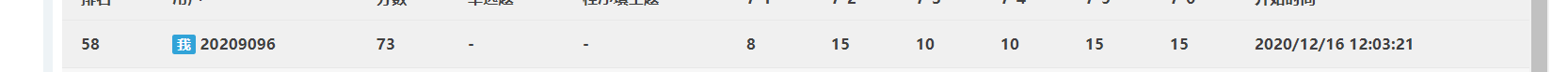### 1.1 题目名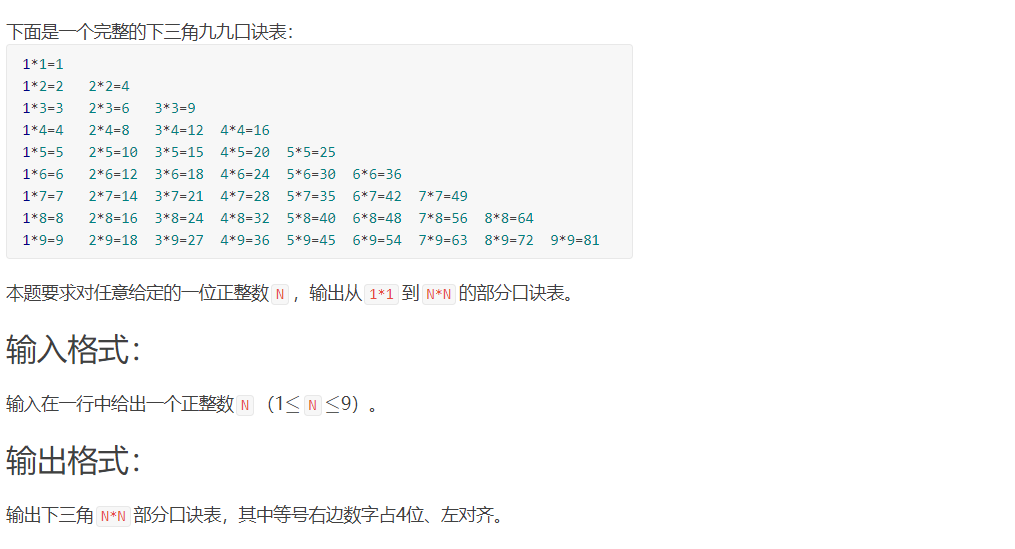1.1.1数据处理

1.1.2实验代码截图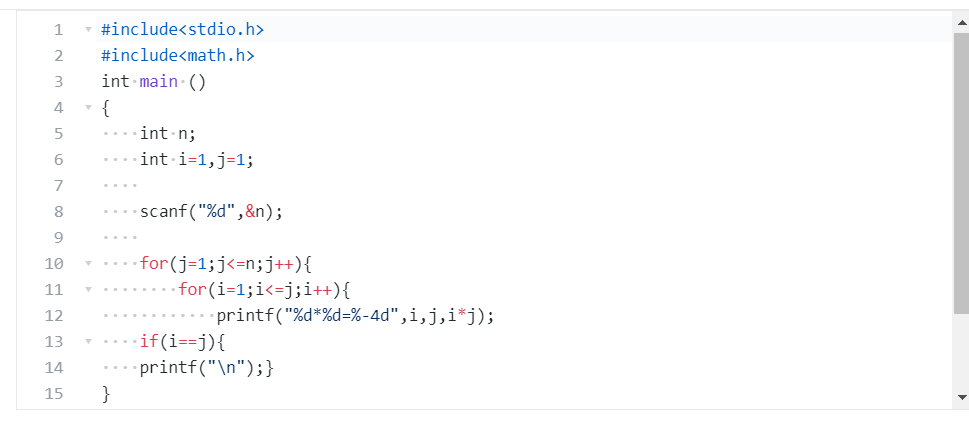1.1.3 造测试数据

5 1 1=1 ， 1 2=2 ， 2 2=4 ， 1 3=3 ， 2 3=6 ， 3 3=9， 1* 4=4 ， 2 4=8 ， 3 4=12 ， 4* 4=16 ， 1* 5=5 ， 2 *5=10 ， 3 * 5=15 ， 4 * 5=20， 5 *5=25 测试值
4 1 1=1 ， 1 2=2 ， 2* 2=4 ，1* 3=3 ，2* 3=6 ， 3* 3=9， 1* 4=4 ， 2 *4=8 ， 3 *4=12 ， 4 *4=16 正确

1.1.4 PTA提交列表及说明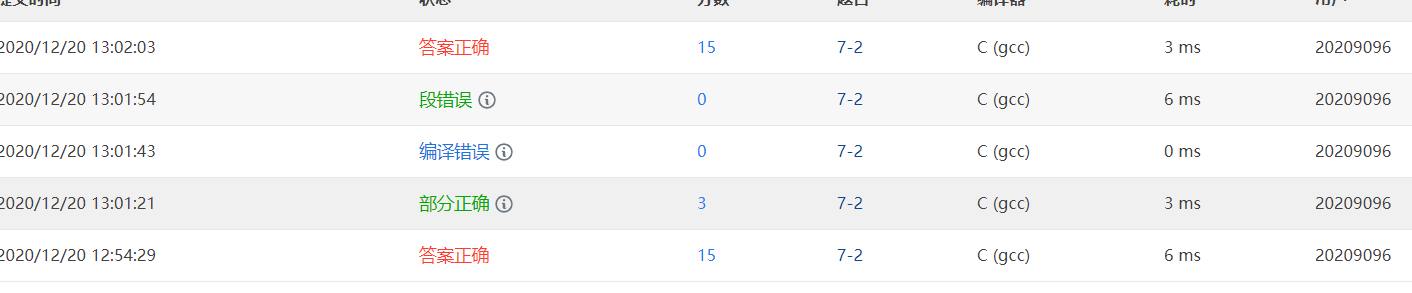### 1.2 题目2

1.2.1 数据处理

1.2.2 实验代码截图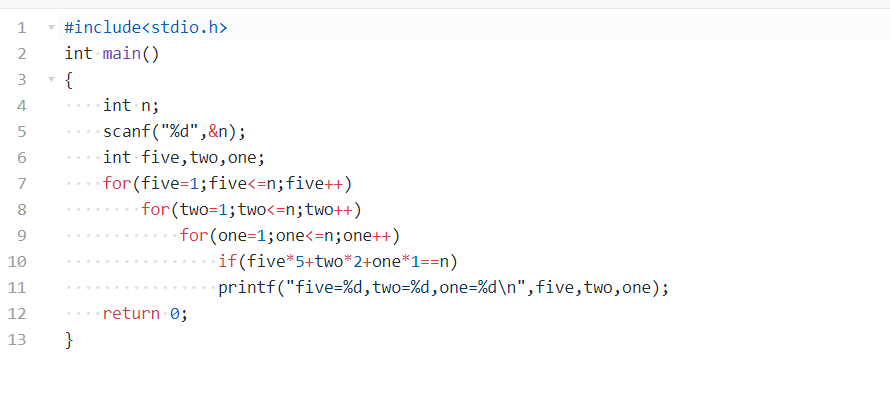1.2.3 造测试数据

13 five=1,two=1,one=6 five=1,two=2,one=4 five=1,two=3,one=2 five=2,two=1,one=1 正确
10 five=1,two=1,one=3 five=1,two=2,one=1 测试数据

1.2.4 PTA提交列表及说明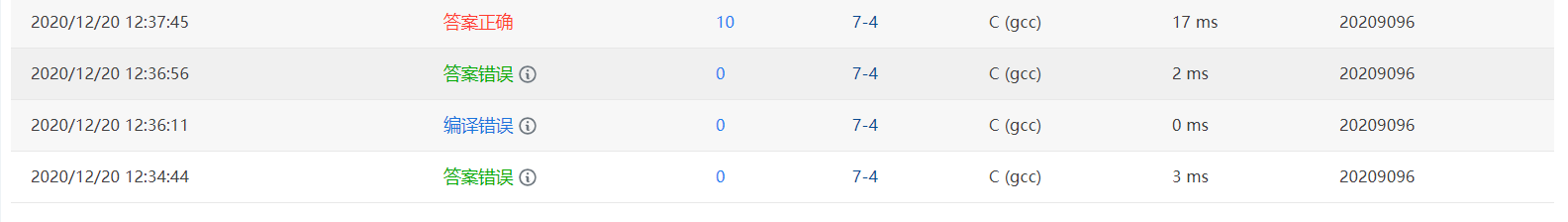## 2.代码互评（5分）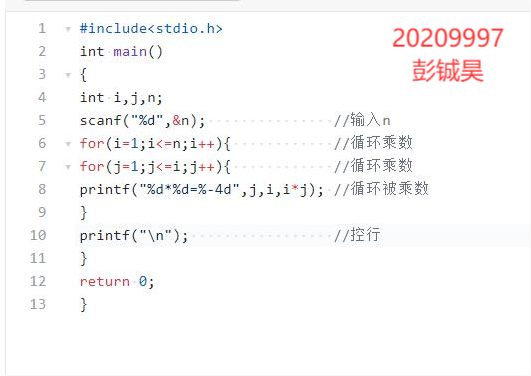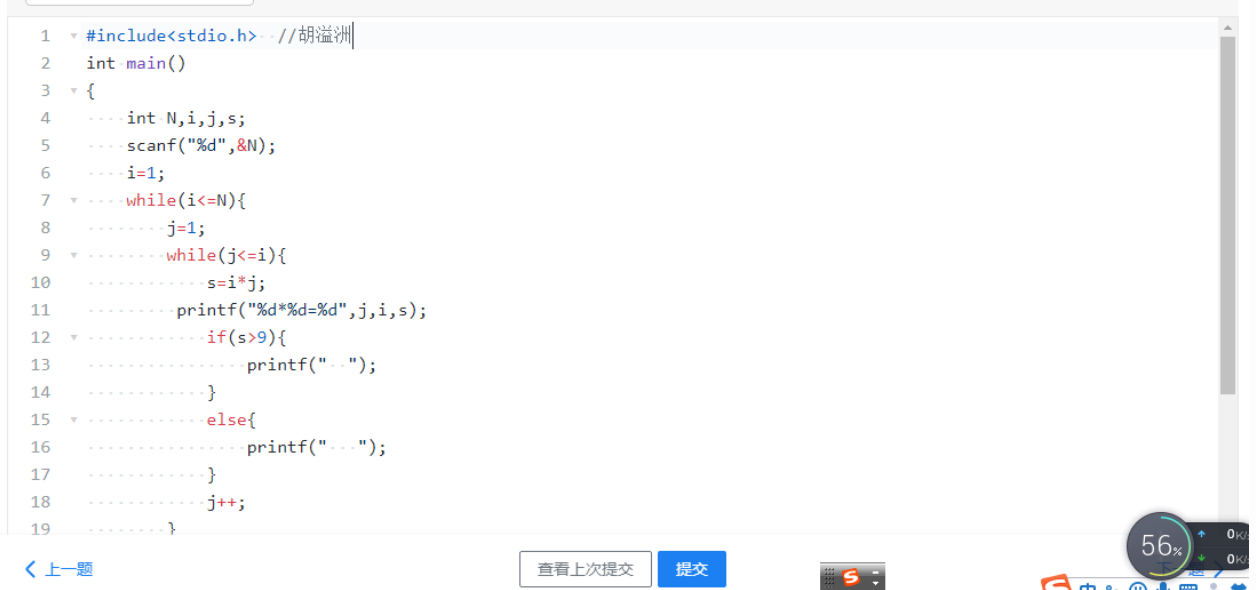（1）同学a和同学b的代码有很大的差别，但他们都值得我学习，第一位同学对前面的学习很扎实。这是我没有的，也是值得我学习的。
（2）我们三个的代码都有不同的地方，第一位同学使用了if-else语句而后面一位同学则有后面的解释。

## 3.学习总结

3.1 学习进度条

3.2 累积代码行和博客字数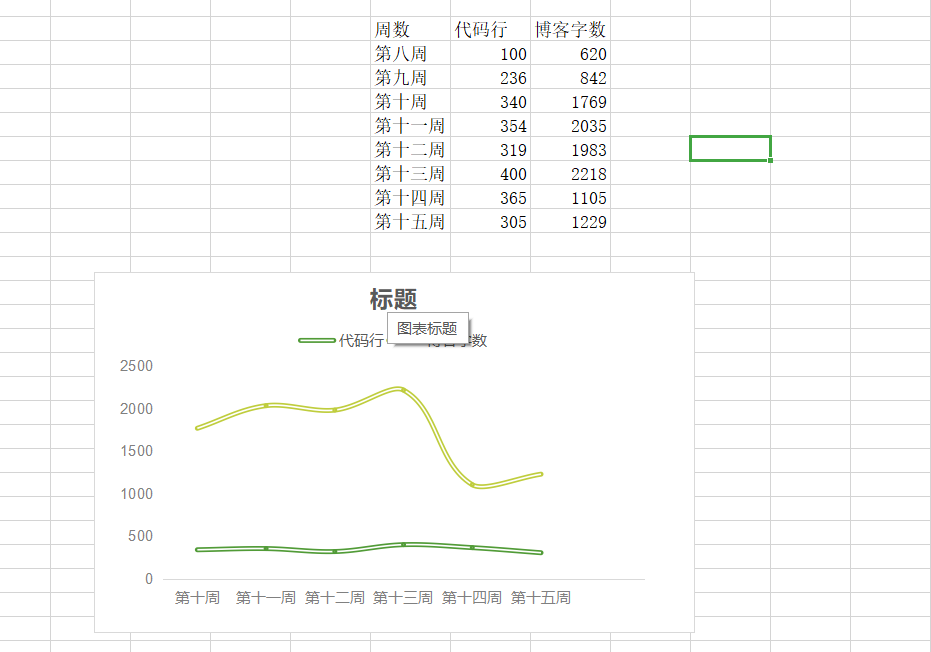3.3 学习内容总结和感悟
3.3.1 学习内容总结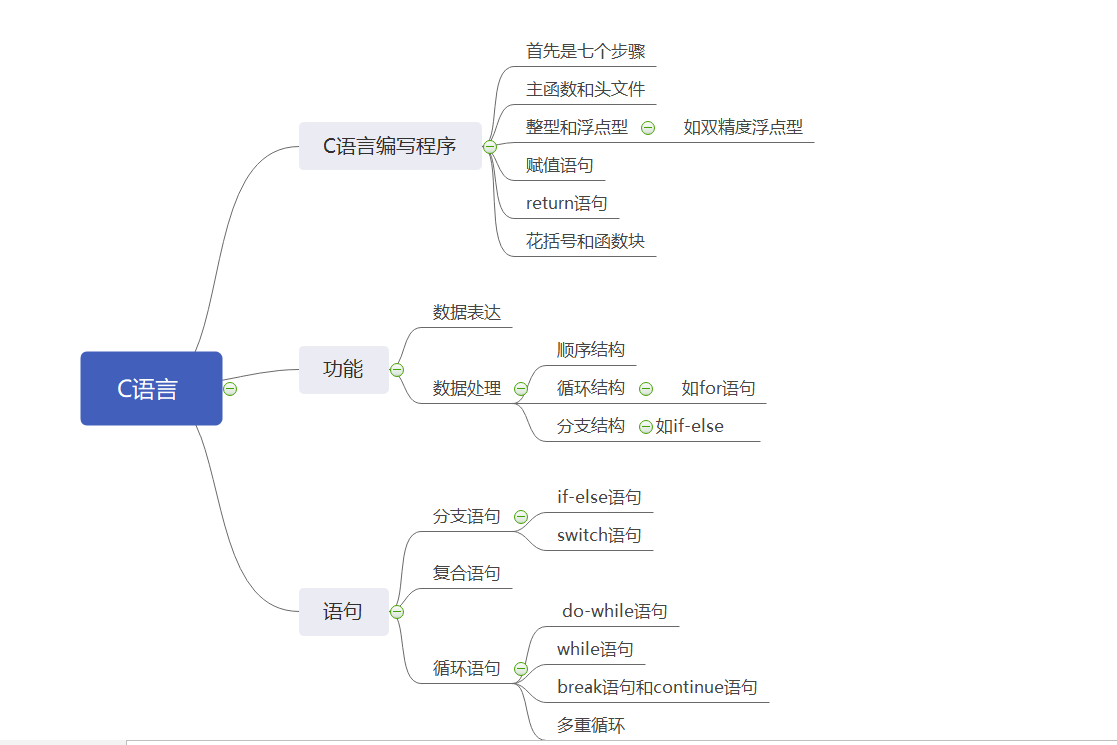3.3.2 学习体会

(1)使用goto
（2）写成函数return
（3）直接修改外层循环条件
（4）在外层循环设置判断条件
（5）在大循环后面判断标志加入beak

posted @ 2020-12-20 16:13  陈文杰  阅读(60)  评论(5编辑  收藏  举报